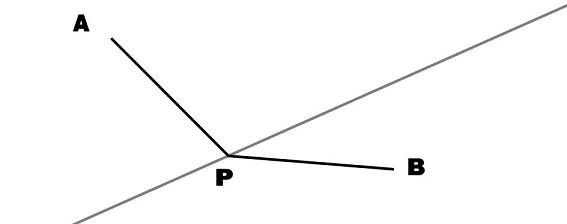#### You may also like### Triangle Midpoints

You are only given the three midpoints of the sides of a triangle. How can you construct the original triangle?### Pareq Exists

Prove that, given any three parallel lines, an equilateral triangle always exists with one vertex on each of the three lines.### The Medieval Octagon

Medieval stonemasons used a method to construct octagons using ruler and compasses... Is the octagon regular? Proof please.

# Two Points Plus One Line

##### Age 14 to 16Challenge Level

Draw a line (considered endless in both directions), put a point somewhere on each side of the line. Label these points $A$ and $B$.

Use a geometric construction to locate a point, $P$, on the line, which is equidistant from $A$ and $B$.Can this point $P$ always be found for any position of $A$ or $B$ ?

If you believe that this is true how might you construct a proof?

If it is false, identify the circumstances when no point $P$, equidistant from $A$ and $B$, exists.

Draw a new arrangement of one line and two points, one on each side of the line.

Imagine creating a collection of similar arrangements.

Can you suggest useful parameters which would uniquely define or identify each arrangement?

Parameters are the numbers (measurements or ratios) you might communicate to another person, say over the telephone, if you wanted them to produce exactly the same arrangement as your own.

These parameters define each arrangement.

Mark a point $O$ somewhere on the line and express the length $OP$ in terms of your parameters.